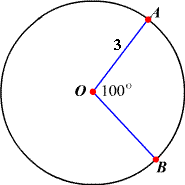# Finding Arc Lengths

There are two ways to measure a circular arc. One is to give its degree measure, which is related to its central angle . For example, in the figure shown, the minor arc $\stackrel{⌢}{AB}$ is formed by a central angle $\angle AOB$ which has measure $100°$ . So we say $m\stackrel{⌢}{AB}=100°$ .To find the length of an arc $\stackrel{⌢}{AB}$ , find the circumference of the circle , and then multiply by the fraction $\frac{m\stackrel{⌢}{AB}}{360°}$ . So for a circle with radius $r$ , the arc length is

$2\pi r\cdot \frac{m\stackrel{⌢}{AB}}{360}$ .

For example, in the circle above, the radius is $3$ units and the measure of the angle is $100°$ . So the length of $\stackrel{⌢}{AB}$ is